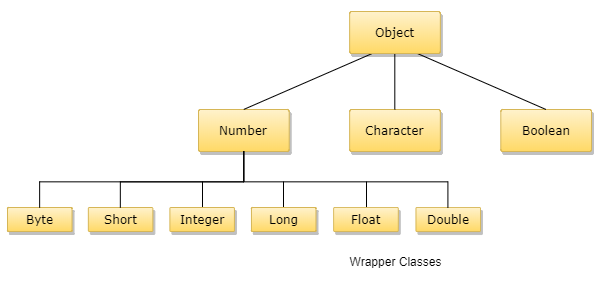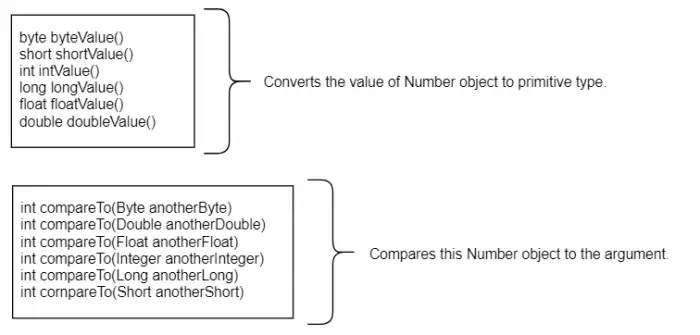# Java Numbers

In most of the cases, programmers use the primary data types such as byte, int, long, double, etc. to work with numbers. This is because these are most commonly used data types which come in handy while creating usual programs.

Some of these are:

``````int g = 260;

float cgpa = 9.14;``````

But there may arise some situations where the programmers have to deal with objects rather than the fundamental data types used on a regular basis. To achieve such scenarios programmers' have to use the wrapper class. Each of Java's primary data types has a class devoted to it termed as a wrapper class. These are termed so because they can wrap up the fundamental data types into an object, of such classes. These wrapper classes come under the java.lang package that gets usually imported as a default package for every Java program.

The various wrapper classes which provide additional power to numbers in Java are:

• Integer
• Short
• Byte
• Float
• Long
• Double

All of these wrapper classes come under of the umbrella of abstract class Number.

A hierarchical view of these wrapper classes are:### Autoboxing and Unboxing

The automatic conversion done by Java compiler for making a between the primitive types with their equivalent object wrapper classes is termed as Autoboxing. We can take the basic example of converting an int -> Integer or a float -> Float. In other words, the conversion from primary data type to their respective Wrapper class objects is called boxing and is done entirely by the compiler without programmer's intervention.

The conversion back from Wrapper object to primary data type, i.e., reversing of Autoboxing mechanism is known as Unboxing.

Here's a simple example of how automatically this Autoboxing and Unboxing takes place:

Example Program:
``````public class Example
{
public static void main(String argu[]) {
Integer g = 6; // autoboxing of int to Integer wrapper object
System.out.println(g);
g =  g - 2;   // unboxing done, where the Integer object value back to int
System.out.println(g);
}
}``````

Here are the lists of instance methods used by all subclasses of Number class:### Methods and Their Uses

• compareTo(): The approach compares the Number item that invoked the method to the argument. It is possible to examine Byte, Long, Integer, and so on
• equals(): This technique determines whether the Number object that invokes the method is identical to the object that is handed as an argument
• valueOf(): The valueOf method usually returns a relevant Number Object conserving the cost of the argument passed. The arguments are of primitive data type or String. This method provides static approach. The approach can take two arguments; wherein one can be String while the other is a radix.
• toString(): The method is used for getting a String object representing the value of the Number Object.
• abs(): The method offers the absolute value of any given argument. The argument can be any of the listed primitive type - int, float, long, double, short or byte.
• ceil(): The ceil() method provides the smallest integer by transforming, which is greater than or equal to the specified argument.
• floor(): The floor() method provides the largest integer by transforming, which is less than or equal to the specified argument.
Example Program:
``````public class Multi_Example {

public static void main(String argu[]) {
Integer g = 6;
Integer d = 4;
Double c = Double.valueOf(5);
Float fl = Float.valueOf("62");
System.out.println(g.compareTo(6));
System.out.println(g.compareTo(4));

System.out.println(g.equals(d));
System.out.println(c);
System.out.println(fl);

Integer h = 22;
System.out.println(h.toString());
System.out.println(Integer.toString(22));

Integer val = -5;
System.out.println(Math.abs(val));

double val2 = -200.456;
System.out.println(Math.ceil(val2));
System.out.println(Math.floor(val2));
}

}``````
Program Output:
```0
1
false
5.0
62.0
22
22
5
-200.0
-201.0```